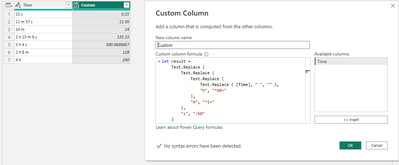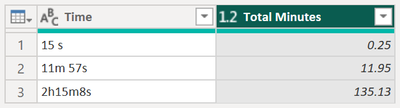cancel
Showing results for
Did you mean:Regular Visitor

## Convert number with text to number

Hello all, I have a Duration column on a report that I need to transform into total minutes.

The current outputs are a variation of the follow three formats:

15 s

11 m 57 s

2 h 15 m 8 s

I really only need total minutes, but would be fine with a format that can average the total time in h:m:s. I'm still learning Power BI and would appreciate some guidance.

1 ACCEPTED SOLUTIONCommunity Support

Hi @SenoirB

I modified @AntrikshSharma 's solution as below. You can use this code to create a custom column.

``````let result =
Text.Replace (
Text.Replace (
Text.Replace (
Text.Replace ( [Time], " ", "" ),
"h", "*60+"
),
"m", "*1+"
),
"s", "/60"
)
in
if Text.EndsWith(result, "+")
then Expression.Evaluate(Text.Range(result, 0, Text.Length(result)-1))
else Expression.Evaluate(result)``````Best Regards,
Community Support Team _ Jing
If this post helps, please Accept it as Solution to help other members find it.

9 REPLIES 9Community Champion

Here is another way to solve this:

``````Expression.Evaluate (
Text.Replace (
Text.Replace (
Text.Replace (
Text.Replace ( [Time], " ", "" ),
"h", "*60+"
),
"m", "*1+"
),
"s", "/60"
)
) ``````Regular Visitor

This one was really close but I get an error if there isn't a "s" on the value. Very few of the entries have a value that looks like this:   14 m

I didn't know these were on the there without seeing the errors from the Custom Column. I tried to come up with a solution by Splitting the column and replacing values and couldn't.Community Support

Hi @SenoirB

I modified @AntrikshSharma 's solution as below. You can use this code to create a custom column.

``````let result =
Text.Replace (
Text.Replace (
Text.Replace (
Text.Replace ( [Time], " ", "" ),
"h", "*60+"
),
"m", "*1+"
),
"s", "/60"
)
in
if Text.EndsWith(result, "+")
then Expression.Evaluate(Text.Range(result, 0, Text.Length(result)-1))
else Expression.Evaluate(result)``````Best Regards,
Community Support Team _ Jing
If this post helps, please Accept it as Solution to help other members find it.Regular Visitor

This worked perfectly! Thank you!Responsive Resident

To transform the Duration column into total minutes in Power BI, you can follow these steps:

1. Create a new column: Right-click on the table, select "Add Column" -> "Custom Column".
2. In the formula bar, use the following formula to extract the values of seconds, minutes, and hours from the Duration column:

= let
durationList = Text.Split([Duration], " "),
durationSeconds =
if List.Count(durationList) = 1 then
Number.FromText(durationList{0})
else if List.Count(durationList) = 3 then
Number.FromText(durationList{0}) * 3600 +
Number.FromText(durationList{1}) * 60 +
Number.FromText(durationList{2})
else if List.Count(durationList) = 4 then
Number.FromText(durationList{0}) * 86400 +
Number.FromText(durationList{1}) * 3600 +
Number.FromText(durationList{2}) * 60 +
Number.FromText(durationList{3})
else null
in
durationSeconds
3. This formula splits the Duration column into a list of values, and then calculates the total seconds by multiplying the hours by 3600, the minutes by 60, and adding up the seconds. If the Duration is in seconds or days, it returns the appropriate value.
4. After creating the new column, you can change the data type to "Duration" or "Time" and format it to display in minutes.
5. You can also create a new measure to average the total time in h:m:s:
Average Time = AVERAGE('Table'[Duration in Minutes])Regular Visitor

Hello @Adamboer thank you for the reply. I tried adding your formula to a custom column and I get errors for results. The row is called Duration so I'm not sure? Does the formula account for the letter "h", "m", "s" that are in the values?Regular Visitor

Thank you for the response. I may have left out some details. I copy and paste the data into Sharepoint, so the report's data source is from Sharepoint. I was entering your code in a Custom Column formula, I don't think that is correct either because it was creating a Table in the Added Column for each entry. Sorry I am really new to Power Queries and Power BI. But your result in Total Minutes is exactly what I am after.Community Champion

@SenoirB My code is query so it will return a table, you need to use the AddedCustom step and create a new step in your code. Otherwise just paste the whole code in a blank query and you can see how it works.Community Champion

@SenoirB Try this:

``````let
Source = Table.FromRows(Json.Document(Binary.Decompress(Binary.FromText("i45WMjRVKFaK1QEyDHMVTM0hbKMMQ9NcCyA7FgA=", BinaryEncoding.Base64), Compression.Deflate)), let _t = ((type nullable text) meta [Serialized.Text = true]) in type table [Time = _t]),
ChangedType = Table.TransformColumnTypes ( Source, { { "Time", type text } } ),
ChangedType,
"Total Minutes",
each
let
RemoveSpace = Text.Replace ( [Time], " ", "" ),
Split = Splitter.SplitTextByCharacterTransition ( { "a" .. "z" }, { "0" .. "9" } ) ( RemoveSpace ),
Transform =
List.Transform (
Split,
each
let
Number = Number.From ( Text.Select ( _, { "0" .. "9" } ) ),
Correction =
if Text.Contains ( _, "h" ) then Number * 60
else if Text.Contains ( _, "m" ) then Number * 1
else if Text.Contains ( _, "s" ) then Number / 60
else null
in
Correction
)
in
Number.Round ( List.Sum ( Transform ), 2 ),
type number
)
in